# Angular Acceleration

## Introduction

Angular acceleration is a concept of rotational dynamics just as acceleration is defined in the linear case. Physically, it describes the rate of change of angular velocity of a particle. Since rotational motion is performed about an axis or a point, angular acceleration has different values depending upon the choice of our origin.

Angular acceleration may be caused by the application of an external torque, or it can be brought about due to changes in the configuration of a body without any external effects. A common example of the latter case is when a person sitting in a revolving chair gains angular velocity when they pull their arms towards themselves.

## What is Angular Acceleration?

Angular acceleration is the rotational analogue of linear acceleration. It is mathematically defined as the time derivative of angular velocity and is commonly represented by the Greek alphabet alpha (α). The angular acceleration is a vector quantity, with a direction normal to the plane of motion of the particle.

## Angular Acceleration Unit

We know that the angular position of an object is measured in radians, and thus, angular velocity is measured in radians per second. Since angular acceleration is the rate of change of this quantity, its unit can be readily derived.

Thus, in the SI system, the unit of angular acceleration is radians per second squared (rad/s2), which corresponds to the dimensions $\mathrm{T^{−2}}$.

## Angular Acceleration Formula

Consider an object whose angular position and angular velocity are represented by theta $\mathrm{(\theta)}$ and omega $\mathrm{(\omega)}$. The simplest formula for angular acceleration is given as the time derivative of angular velocity.

$$\mathrm{\alpha=\frac{d\omega}{dt}}$$

Further, since angular velocity itself depends upon angular position, we also have

$$\mathrm{\alpha=\frac{d^2\theta}{dt^2}}$$

The angular acceleration can also be estimated by dividing the difference between final and initial angular velocities to the corresponding change in time.

$$\mathrm{\alpha=\frac{\omega_2-\omega_1}{t_2-t_1}=\frac{\Delta \omega}{\Delta t}}$$

## Angular Acceleration Examples

1. A stone whirled on a taut string achieves an angular velocity of 30 rad/s in 15 seconds. Find the angular acceleration of the stone.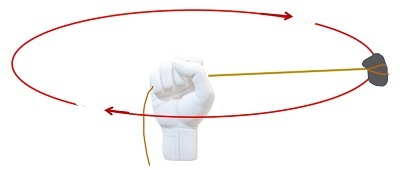Answer: Given that it takes 15 seconds for the stone to achieve an angular velocity of 30 rad/sec.

$$\mathrm{\alpha=\frac{\Delta \omega}{\Delta t}=\frac{30-0}{15-0}=\frac{30}{15}=2\:rad/sec^2 }$$

2. The angular rotation of an object is given by the equation $\mathrm{\theta=3t^4+6t^2-7t+2}$. Find its angular acceleration at t=7sec.

$$\mathrm{\alpha=\frac{d^2\theta}{dt^2}}$$

$$\mathrm{\alpha=\frac{d^2}{dt^2}(3t^4+6t^2-7t+2)=36t^2+12}$$

At t=7 sec

$$\mathrm{\alpha=36×(7)^2+12=1776\:rad/s2}$$

## Application of Angular Acceleration

### Wheel of an Accelerating Car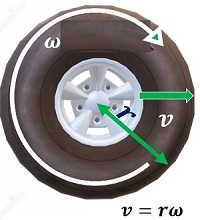When we accelerate or decelerate our car, the angular velocity of the wheels increases or decreases accordingly. That is, the wheels receive an angular acceleration that is connected to the linear acceleration of the car by the formula

$$\mathrm{\alpha=\frac{a}{r}}$$

### Ceiling FansA ceiling fan starts slow but quickly reaches its desired speed. That is, its angular velocity increases before reaching a certain value. Hence, an angular acceleration is associated with its motion. Note that since the fan is spinning about its axis, this is an example of spin angular acceleration, just like the previous example.

## Types of Angular Acceleration

There are two types of motions possible under rotational motion.

### Spin Angular Acceleration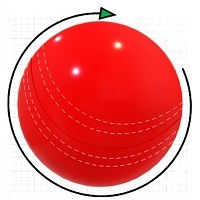A rigid object can rotate about an axis that passes through it, giving rise to what is known as the spin of the particle. A common example of this is the spinning ball in cricket.

The angular acceleration associated with this type of motion is known as the spin angular acceleration.

### Orbital Angular Acceleration

On the other hand, any object, rigid or otherwise, can execute motion about a point, giving rise to an orbital motion of sorts. The easiest way to imagine this motion is to look at planetary motion. All planets revolve around the sun in elliptical orbits and thus, possess an orbital motion. Such type of motion involves orbital angular acceleration.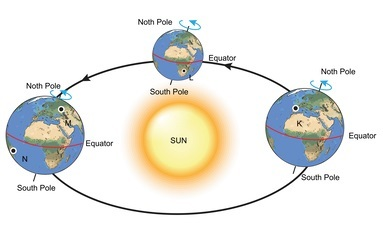It is also possible for an object to have both spin as well as orbital motion. The simultaneous rotation and revolution of the Earth is a very simple example of such a scenario.

## Orbital Angular Acceleration of a Point Particle: Two-Dimensions

In two-dimensional case, when a body is in motion with respect to a point, it can possess an angular acceleration. This is referred to as its orbital angular acceleration and is equal to the instantaneous rate of change of the orbital angular velocity.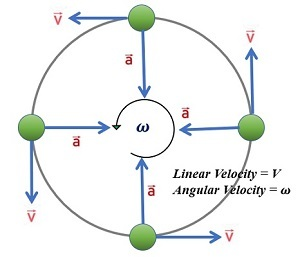The green arrow in this diagram represents the tangential component of the particle’s instantaneous velocity, while the purple one represents angular velocity.

$$\mathrm{v=r\omega}$$

$$\mathrm{\omega=\frac{v}{r}}$$

We know that

$$\mathrm{\alpha=\frac{d\omega}{dt}=\frac{d}{dt}(\frac{v}{r})}$$

To solve this, apply the product rule of differential calculus. We will get

$$\mathrm{\alpha=\frac{1}{r}\frac{dv}{dt}=\frac{v}{r^2}(\frac{dr}{dt})}$$

This is the orbital angular acceleration of a point particle in two dimensions.

Special case: If a particle is executing circular motion, which means that the radius remains constant in time. So angular acceleration is written as

$$\mathrm{alpha=\frac{1}{r}\frac{dv}{dt}}$$

## Relation between Angular Acceleration and Linear Acceleration

We again start by deriving the formula for angular acceleration. To do so, consider an object moving with linear velocity v. Then, the angular velocity is

$$\mathrm{\omega=\frac{v}{r}}$$

Therefore,

$$\mathrm{\alpha=\frac{d\omega}{dt}=\frac{d}{dt}(\frac{v}{r})}$$

Using product rule,

$$\mathrm{\alpha=\frac{1}{r}\frac{dv}{dt}=\frac{v}{r^2}(\frac{dr}{dt})}$$

When the radius of the particle is not constant, the relation between angular and linear acceleration is difficult to formulate.

However, when the radius remains invariant,

$$\mathrm{\frac{dr}{dt}=0}$$

$$\mathrm{\alpha=\frac{1}{r}\frac{dv}{dt}}$$

Hence,

$$\mathrm{\alpha=\frac{a}{r}}$$

## Angular Acceleration Formula

Angular acceleration of an object may be obtained by any of the following formulae −

$$\mathrm{\alpha=\frac{d\omega}{dt}=\frac{d^2\theta}{dt^2}}$$

$$\mathrm{\alpha=\frac{1}{r}\frac{dv}{dt}-\frac{v}{r^2}\frac{dr}{dt}}$$

When the motion is circular,

$$\mathrm{\alpha=\frac{1}{r}\frac{dv}{dt}=\frac{a}{r}}$$

## Solved Questions

Q1. An object’s angular velocity changes from $\mathrm{\frac{\pi}{4}}$ rad/s to $\mathrm{\frac{3\pi}{8}}$ rad/s in 0.75 seconds. Find its angular acceleration.

Ans. Using

$$\mathrm{\alpha=\frac{\Delta\omega}{\Delta t}}$$

we have

$$\mathrm{\alpha=\frac{\frac{3\pi}{8}-\frac{\pi}{4}}{0.75}=\frac{\frac{\pi}{8}}{0.75}=\frac{\pi}{6}\:rad/s^2}$$

Q2. The angular acceleration of a particle is 3 rad/s2. If it starts from rest, find its angular velocity after it has covered a distance of 46 radians.

Ans. Let $\mathrm{\omega_1}$ and $\mathrm{\omega_2}$ be the initial and final angular velocities of the particle, and θ be the distance covered.

The equation of motion is $\mathrm{\omega_2^{2}-\omega_2^{1}=2\alpha \theta}$

Here, the particle starts from rest and thus, $\mathrm{\omega_1=0}$.

Therefore,

$$\mathrm{\omega_2=\sqrt{2\alpha \theta}=\sqrt{2\times 3\times46}=\sqrt{276}=16.61 rad/s.}$$

## Conclusion

Angular acceleration is the rotational analogue of linear acceleration and is given by the rate of change of angular velocity. Its value changes depending on the choice of origin and thus, even particles in straight line motion can possess angular acceleration. For circular motion, angular acceleration is given by $\mathrm{\alpha=\frac{1}{r}\frac{dv}{dt}=\frac{a}{r}}$ The equations of motions are equally valid for the rotational case as they are for the linear case.

## FAQs

Q1. Does a particle in straight line motion possess angular acceleration?

Ans. Yes. Depending on the origin chosen, the angular velocity of the particle may not be constant and thus, there is a possibility of angular acceleration.

Q2. Are the equations of motion valid for rotational dynamics?

Ans. Yes, with slight modifications. The equations of motion for the rotational case are given as follows. Note that for these equations to hold true, the angular acceleration must be uniform.

• $\mathrm{\omega_2^{2}-\omega_1^{2}=2\alpha\theta}$

• $\mathrm{\omega_2=\omega_1+\alpha t}$

• $\mathrm{\theta=\omega_1 t+\frac{1}{2} \alpha t^2}$

Q3. What is the direction of angular acceleration?

Ans. Angular acceleration vector points in the direction normal to the plane of motion. Its sign is positive if the angular velocity is increasing in the counter clockwise direction and negative otherwise.

Q4. Does the Earth possess any angular acceleration?

Ans. The angular acceleration of Earth is almost zero about its axis. There are a variety of factors that cause infinitesimally small amounts of changes to the Earth’s angular velocity, but these are too small when compared to the actual angular velocity of the earth (about 2π radians per day).

Q5. What is the orbital angular acceleration when a particle is moving in three dimensions?

Ans. In three dimensions, the angular velocity is given as

$$\mathrm{\omega=\frac{r×v}{r^2}}$$

On differentiating with respect to time, we get

$$\mathrm{\alpha=\frac{r\times a}{r^2}-\frac{2}{r}\frac{dr}{dt}\omega}$$

Q6. What is the angular acceleration of a simple pendulum?

Ans. For a simple pendulum, the equation of motion reads $\mathrm{\ddot{\theta}=-\frac{g}{R}\:sin\:\theta}$ . This is the value of the angular acceleration experienced by a simple pendulum at an instant where it subtends an angle of θ with the vertical.

Updated on: 06-Apr-2023

250 Views